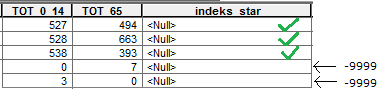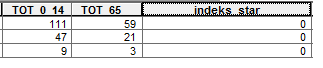# Calculating field values only for appropriate features using ArcMap

1629
14
02-01-2020 10:50 AMOccasional ContributorJust like in the attached picture. I would like to calculate the value for the field "indeks_star" with the formula: indeks_star =[TOT_65__] / [TOT_0_14] * 100

And for features where in the field TOT_0_14 occurs 0 or in [TOT_65__] occurs 0, I would like the value -9999 to appear in the field indeks_star.

What formula to enter in the field calculator to simultaneously calculate all values for the field indeks_star?

Tags (3)
1 Solution

Accepted SolutionsbyMVP Frequent Contributor

Hello again,

on field calculator, select Python as your parser (top radio button) and select 'show codeblock' which appears in the middle of the window.

where it has whitespace below 'pre-logic script code' insert the code below

``````def percentage(field1, field2) :

if field1 != 0 and field2 != 0 :
return ( (float(field1) / float(field2) ) * 100.0)

else :
return -9999‍‍‍‍‍‍‍‍‍‍‍‍‍‍‍‍‍‍‍‍‍``````

in the bottom bit of whitespace it should have something like 'indeks_star =' above it in the greyed out area.  in the whitespace type out this:

percentage(!TOT_0_14!, !TOT_65!)

14 RepliesbyMVP Frequent Contributor

Hello again,

on field calculator, select Python as your parser (top radio button) and select 'show codeblock' which appears in the middle of the window.

where it has whitespace below 'pre-logic script code' insert the code below

``````def percentage(field1, field2) :

if field1 != 0 and field2 != 0 :
return ( (float(field1) / float(field2) ) * 100.0)

else :
return -9999‍‍‍‍‍‍‍‍‍‍‍‍‍‍‍‍‍‍‍‍‍``````

in the bottom bit of whitespace it should have something like 'indeks_star =' above it in the greyed out area.  in the whitespace type out this:

percentage(!TOT_0_14!, !TOT_65!)Occasional Contributor

shouldn't it be the other way around?

no
percentage(!TOT_0_14!, !TOT_65!)         but      percentage( !TOT_65!, !TOT_0_14!)byMVP Frequent Contributor

Depends which way round you want it really, in your question you wanted _14/_65 ?Occasional Contributor

I just edited the post now. Sorry for the mistake. Should be [TOT_65__] / [TOT_0_14] * 100

so it will be          percentage( !TOT_65__!, !TOT_0_14!)      ?byMVP Frequent Contributor

Yeh should workOccasional Contributor

many features calculated correctly, many -9999(no problem), but there are also many cases when the value of the field indeks_star should be calculated, but a value of 0 for this field is created.byMVP Frequent Contributor

Maybe it's doing integer division. I'll amend the code nowOccasional Contributor

all values seem fine now. thank you!!!I have to create one more field and calculate one more formula, will you wait 5 minutes? I'm already putting it hereOccasional ContributorAProchow = (TOT_65/TOT)*100

if field TOT is 0 or field TOT_65__ is 0   then field  AProchow equals -9999